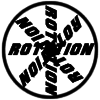#### You may also like### 8 Methods for Three by One

This problem in geometry has been solved in no less than EIGHT ways by a pair of students. How would you solve it? How many of their solutions can you follow? How are they the same or different? Which do you like best?### Rots and Refs

Follow hints using a little coordinate geometry, plane geometry and trig to see how matrices are used to work on transformations of the plane.### Reflect Again

Follow hints to investigate the matrix which gives a reflection of the plane in the line y=tanx. Show that the combination of two reflections in intersecting lines is a rotation.

# Transformations for 10

##### Age 16 to 18Challenge Level

The equations for a line and plane in vector form may be useful.

Line: ${\bf r}={\bf a} + \lambda{\bf b}$
Plane: ${\bf r}={\bf a} + \lambda{\bf b}+ \mu{\bf c}$

It may also be useful to recall that matrix multiplication is distributive:
${\bf M}({\bf a} + {\bf b}) = {\bf Ma} + {\bf Mb}$

This problem uses concepts met in the later Further Pure Maths A level modules.